# climpred.metrics._pearson_r_eff_p_value#

climpred.metrics._pearson_r_eff_p_value(forecast: xarray.Dataset, verif: xarray.Dataset, dim: Optional[Union[str, List[str]]] = None, **metric_kwargs: Any) [source]#

pearson_r_p_value accounting for autocorrelation.

Note

Weights are not included here due to the dependence on temporal autocorrelation.

Note

This metric can only be used for hindcast-type simulations.

The effective p value is computed by replacing the sample size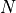in the t-statistic with the effective sample size,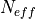. The same Pearson product-moment correlation coefficient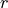is used as when computing the standard p value.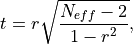whereis computed via the autocorrelation in the forecast and verification data.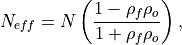where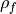and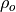are the lag-1 autocorrelation coefficients for the forecast and verification data.

Parameters

Notes

 minimum 0.0 maximum 1.0 perfect 1.0 orientation negative

Example

>>> HindcastEnsemble.verify(
...     metric="pearson_r_eff_p_value",
...     comparison="e2o",
...     alignment="same_verifs",
...     dim="init",
... )
<xarray.Dataset>
Coordinates:
* lead     (lead) int32 1 2 3 4 5 6 7 8 9 10
skill    <U11 'initialized'
Data variables:
SST      (lead) float64 0.02333 0.08552 0.2679 ... 0.2369 0.2588 0.2703
Attributes: